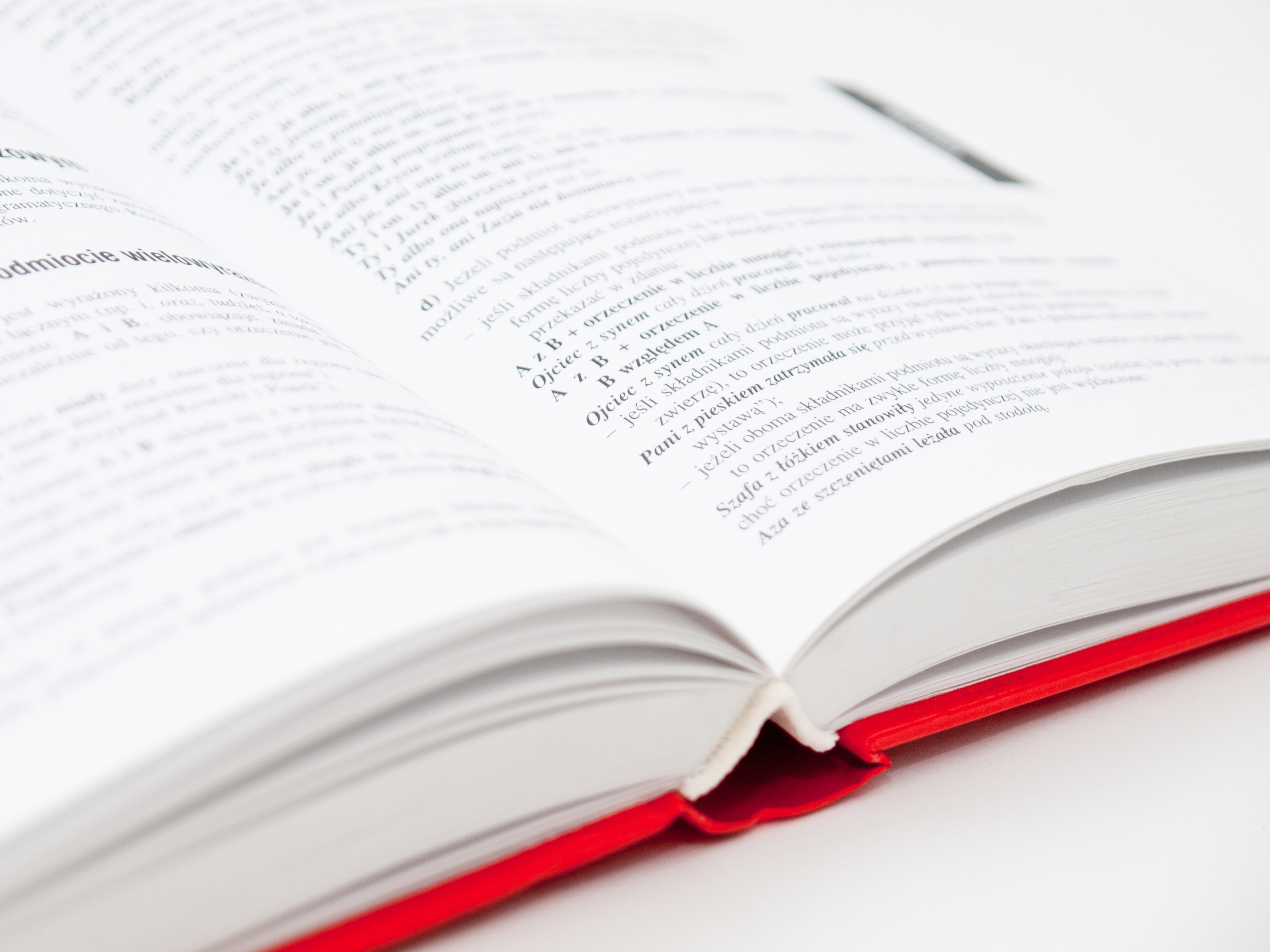# Solution to problems – class 9 – Set 1 Q 18

## Problem Statement

A car starts from rest and accelerates uniformly over a time of 7 seconds for a distance of 98 m. Determine the acceleration of the car.

## Solution

Distance travelled: S = ut + ½ a t2

As u = 0, the equation becomes    S = ½ at2

so, a = 2S/t2 …..(1)

t = 7 seconds
S=98 m

from eqn   1 we get acceleration a = 2S/t2
= (2 * 98)/72 =4 m/s2Scroll to top
error: physicsTeacher.in